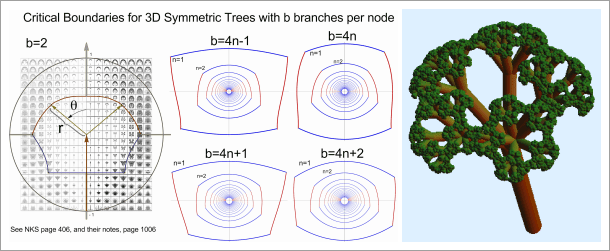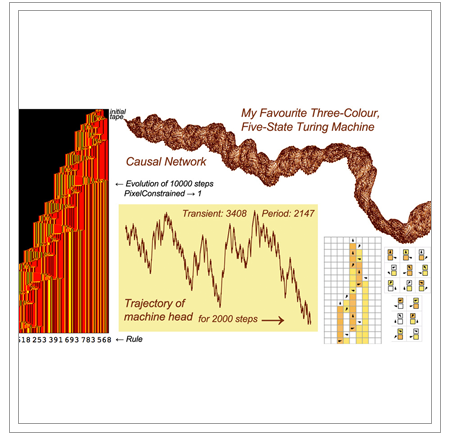#Wolfram Summer School

# Alumni# Bernat Espigulé Pons

Summer School

Class of 2013

## Bio

Bernat Espigulé Pons is the author of a CDF-based website that guides its visitors around the forest of symmetric fractal trees. Equipped with Mathematica, Bernat has discovered and mapped the generalized families of planar self-contacting symmetric fractal trees. His main results have been presented in two papers at the Bridges Conference and the Symmetry Festival 2013. Attempts to characterize the complex behavior found in self-overlapping trees are still in progress and they will require advanced NKS techniques.

In 2012, Bernat received a BSc in physics from Universitat Autónoma de Barcelona, completing the last two years of his studies abroad, first as an EAP student at the University of California, Santa Barbara 2010-2011, and then as an ERASMUS student at Heidelberg Universität, Germany 2011-2012. His strong interest in the study of complex systems started at High School where he developed a research project about Geometry and Nature. From past to present, his interests are: fractal geometry, chaos theory, fractal space-time, complex networks, non-linear phenomena, morphogenesis, dynamical systems, topology, complex oscillations, fractal trees and NKS. Bernat’s other interests are photography, hiking, surfing, couchsurfing, capoeira, architecture, generative art, education and, more recently, the art of 3D printing. He also enjoys receiving feedback from his left-handed twin brother who is doing research in physics, and her younger sister majoring in math.

## Project: Generalized Self-Contacting Symmetric Fractal Trees in 3D

In 1985, Barnsley and Hardin studied a Mandelbrot set whose boundary is piecewise smooth. As was shown later by Stephen Wolfram, the Julia sets associated with this Mandelbrot set can be represented as symmetric binary fractal trees. In 1999, Mandelbrot and Frame independently deduced the boundary equations of this Mandelbrot set from direct observations of the relevant branch-addresses leading to tip-to-tip self-contact in symmetric binary fractal trees. Combining their methods with real-time explorations with Mathematica, Bernat Espigulé has obtained the boundary equations for generalized symmetric fractal trees in 2D, mirror-symmetric trees with b>2 branches per node. Bernat has also developed a three-dimensional unfolding process for Koch-like symmetric fractal trees that transforms them to Lévy-curve fractal trees. These last results and the works of Brown et. al. in three-dimensional fractal trees marked the guidelines for the present project.

My project consisted of two parts, first I developed a family of generators leading to three-dimensional fractal trees that are symmetric around the z-axis, and then I mined the “fractal forest” or parameter spaces of fractal trees to obtain boundary equations where the critical transitions take place (transitions from totally disconnected trees to self-overlapping trees). The relevant branch addresses that ensure self-contact in a fractal tree were obtained through direct Mathematica explorations. I derived the general expressions for these addresses and I imposed the tip-to-tip self-contact to constrain them. This provided me with a set of equalities to deduce the desired boundary equations. The explicit forms of these equations give the values of the self-contacting ratio rsc as function of the vertical angle θ.### Summary of results and conclusions

I identified four kinds of symmetric fractal trees in 3D that are determined by the type of expressions found in their boundary equations, these are trees with number of branches b=4n-1, b=4n, b=4n+1 and b=4n+2 where n takes the integer values from 1 to ∞. Several animations were produced when one walks around the critical boundaries of the parameter space, showing interesting dynamics and topological critical changes for certain angles.

### Future directions

In 3D there are more ways to distribute branches forming symmetric trees. Future directions will explore the critical boundaries of 3D fractal trees with different sets of branches distributed at different angles θ.

## Favorite Four-Color, Four State Turing Machine

Rule 14345618253391693783568APPLY NOW